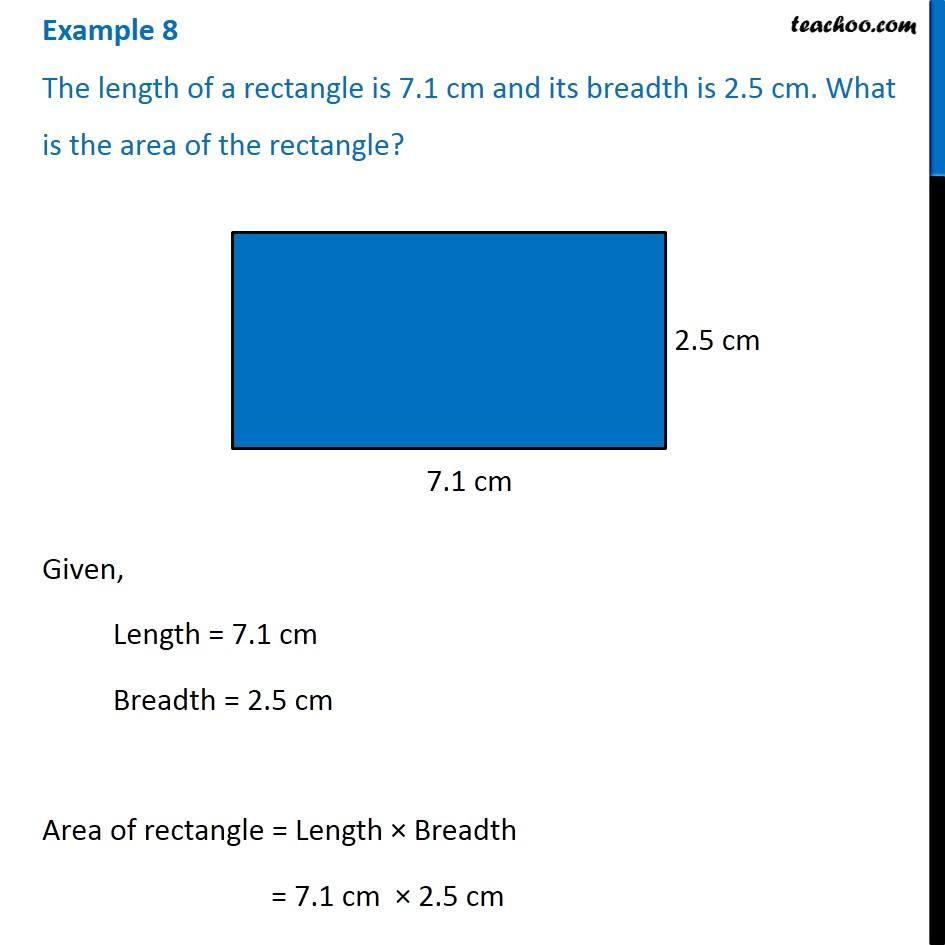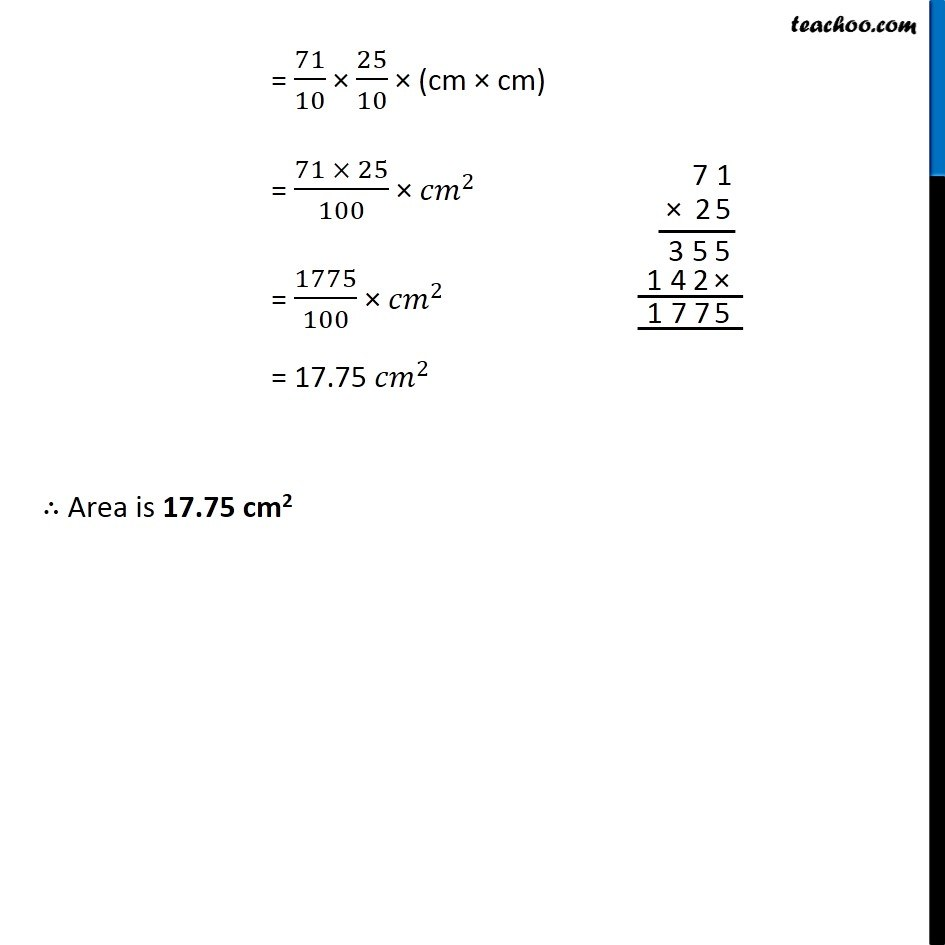Subscribe to our Youtube Channel - https://you.tube/teachoo

1. Chapter 2 Class 7 Fractions and Decimals
2. Concept wise
3. Multiplication of Decimals - Statement Questions

Transcript

Example 8 The length of a rectangle is 7.1 cm and its breadth is 2.5 cm. What is the area of the rectangle?Given, Length = 7.1 cm Breadth = 2.5 cm Area of rectangle = Length × Breadth = 7.1 cm × 2.5 cm = 71/10 × 25/10 × (cm × cm) = (71 × 25)/100 × 〖𝑐𝑚〗^2 = 1775/100 × 〖𝑐𝑚〗^2 = 17.75 〖𝑐𝑚〗^2 ∴ Area is 17.75 cm2

Multiplication of Decimals - Statement Questions